# Wiener measure

Jump to: navigation, search

The probability measureon the space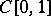of continuous real-valued functionson the interval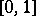, defined as follows. Let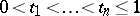be an arbitrary sample of points fromand let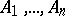be Borel sets on the real line. Let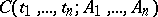denote the set of functions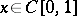for which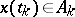,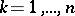. Then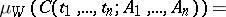(*)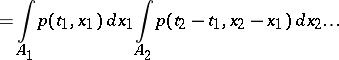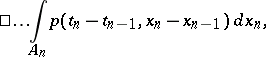where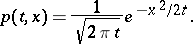Using the theorem on the extension of a measure it is possible to define the value of the measure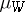on all Borel sets ofon the basis of equation (*).

#### Comments

The Wiener measure was introduced by N. Wiener [a1] in 1923; it was the first major extension of integration theory beyond a finite-dimensional setting. The construction outlined above extends easily to define Wiener measureon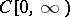. The coordinate process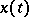is then known as Brownian motion or the Wiener process. Its formal derivative "dxt/dt" is known as Gaussian white noise.